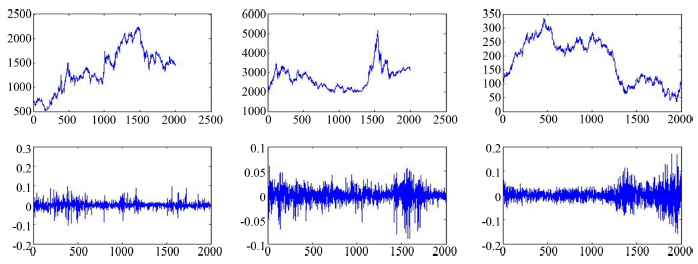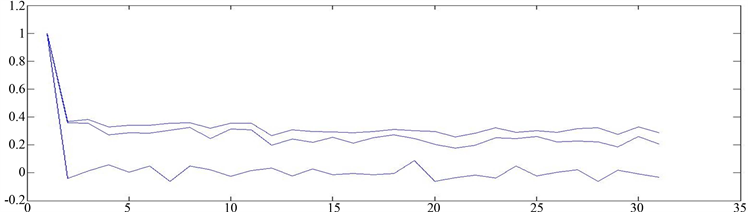﻿ 在做市商机制下预测我国股票市场价格模型的研究

# 在做市商机制下预测我国股票市场价格模型的研究A Study on the Price Forecasting Model of China’s Stock Market under the Market Maker

Abstract: Due to the complexity, dynamics and instability of financial markets, the standard asset pricing model under rational expectations is hard to predict price changes. The expectation beliefs of the fundamentalists are closely related to the price bias in the traditional financial model, however, for chartists, the expectation beliefs are attributed to the learning process of historical prices. Therefore, we set up a simple heterogeneous agent model under the market maker, including two traders (fundamentalists and chartists). This paper studies from the following aspects: 1) The relationship between returns and volatility in China’s stock market is studied; 2) Forecast market price dynamics; 3) Taking the index of Shanghai stock market as the object of study. In this paper, the statistical analysis method is used to compare the simulation results and empirical results of the simulation data obtained from the model, and do statistical tests. The results show that the stock market has high correlation and heteroscedasticity, and volatility and market risk. The returns are asymmetric and there is leverage effect in the market as a whole. This causes price changes. It is found that this model can reflect the real market better.

1. 引言

1.1. 资产价格动态模型研究背景，国内外发展现状及意义

Michele Berardi  建立了一个简单的代表性代理资产定价模型：如果风险厌恶系数随时间变化的，则能够产生程式化事实。此模型很受欢迎是因为他们容易处理以及他们能够产生金融市场中所观察到的著名的程式化事实。众所周知，金融时间序列有共同的特点，所谓的程式化事实，包括过度波动，波动聚集，偏度和过度峰度。传统的经济与金融理论包括代表性代理人和有限预期已经在解释这些程式化事实上遇到了很大的困难，如厚尾，幂律行为(He and Li)  以及收益率的自相关性，波动聚集(Alfarano等)  。传统的计量经济学模型假定样本的方差是保持固定不变，但是随着理论的发展，有些学者发现这样的假设不合理。例如，Mandellbrot  观察发现很多经济变量的方差不断变化，方差的分布具有厚尾性，同时也发现方差在某些时段里变化幅度较大，某些时段里变化幅度较小。恩格尔和克拉格在分析宏观数据时发现时间序列模型中的扰动项方差不满足同方差的假设，经研究发现扰动项的方差取决于后续扰动项平方的大小。于是，Engle  提出了自回归条件异方差ARCH模型，即误差的方差不再是常数，提供了一个分析和产生波动性时间序列模型，也是在金融市场中广泛运用的一种特殊非线性模型，但是它也有局限性，实际上ARCH模型只适用于异方差函数短期的自相关过程，而有些残差序列的异方差函数具有长期自相关性，将会产生很高的移动平均阶数，增加参数的难度，如滞后阶数非常大很复杂的情况以及违反了非负数约束条件等，Bollerslev  将其进行扩展然后发展起来了广义条件自回归(GARCH)模型，后来成为了模拟和预测金融模型波动性最流行的模型，最常用的是GARCH(1,1)模型，GARCH它可以描述大量的金融时间序列数据。由于GARCH类模型不再是通常的线性模型，它能够解释大量的金融数据的主要特征，如尖峰厚尾，波动聚集性和杠杆效应等，我们通常使用最大似然估计以及找到最可能的参数为目的，它以建立对数似然方程来实现，我国股市经过近30年的发展，GARCH模型是一种用过去的方差和过去方差的预测值，来预测未来方差的自回归条件异方差时间序列模型，有着极其广泛的应用，其中异方差是方差随时间而变化的。波动性是金融时间序列数据中最重要的程式化事实之一，以收益的方差或标准差来度量波动性，常用来粗略地测量金融资产的总体风险。历史波动性仅涉及用它作为对所有未来时期的波动性的预测，用GARCH模型等刻画时间序列的波动性。通过非对称性信息曲线分析认为资本市场中的冲击通常表现出非对称效应，波动率对市场下跌的反应比对市场上升时反应更迅速，即所谓的“杠杆效应”。还有些文献研究结果表明如股票价格，利率等经济类时间序列数据经常表现为方差随时间的变化而变化的特点。BM Blau  前面的研究发现，投资者对正偏股票数据的偏好很强，这些偏好已被证明导致价格溢价和随后的表现不佳，这些研究扩展了越来越多的文献，通过是否表现出股票正偏不佳是受投资者高时段情绪，投资者的乐观情绪可能会增加投资者偏斜度偏好。

1.2. 本文的主要工作

2. 异质代理资产价格动态模型的建立

${E}_{f,t}\left({p}_{t+1}\right)={p}_{t}+\alpha \left({p}_{t+1}^{\ast }-{p}_{t}\right)$

${E}_{c,t}\left({p}_{t+1}\right)={p}_{t}+\gamma \left({p}_{t}-{u}_{t}\right)$

${\sigma }_{h,t+1}^{2}=\underset{k=1}{\overset{\infty }{\sum }}{\lambda }_{k}{\left[{E}_{h,t-k}\left({p}_{t-k+1}\right)-{p}_{t-k+1}\right]}^{2},\text{ }\text{ }\text{ }\text{ }\text{ }\text{ }\text{ }\text{ }\text{ }\text{ }h=f,c$

${\sigma }_{f,t+1}^{2}=\left(1-\lambda \right){\sigma }_{f,t}^{2}+\lambda \frac{{\left[{E}_{f,t-1}\left({p}_{t}\right)-{p}_{t}\right]}^{2}}{1+{\left({p}_{t+1}-{p}_{t+1}^{\ast }\right)}^{2}}$

${\sigma }_{c,t+1}^{2}=\left(1-\lambda \right){\sigma }_{c,t}^{2}+\lambda {\left[{E}_{c,t-1}\left({p}_{t}\right)-{p}_{t}\right]}^{2}$

$\left\{\begin{array}{l}{p}_{t+1}={p}_{t}+\frac{\mu }{2}\frac{1+m}{{a}_{1}\left(1+q\right){\sigma }_{1}^{2}}\left[\alpha \left({p}_{t+1}^{\ast }-{p}_{t}\right)-\left(R-1\right)\left({p}_{t}-\stackrel{¯}{p}\right)+\left(1-m\right)\gamma \frac{\left({p}_{t}-{u}_{t}\right)-\left(R-1\right)\left({p}_{t}-\stackrel{¯}{p}\right)}{{a}_{2}{\sigma }_{1}^{2}\left(1+q+b{v}_{t}\right)}\right]+{\delta }_{t}\\ {u}_{t}=\delta {u}_{t-1}+\left(1-\delta \right){p}_{t}\\ {v}_{t}=\delta {v}_{t-1}+\delta \left(1-\delta \right){\left({p}_{t}-{u}_{t-1}\right)}^{2}\\ {p}_{t+1}^{\ast }={p}_{t}^{\ast }\mathrm{exp}\left(-\frac{{\sigma }_{\epsilon }^{2}}{2}+{\sigma }_{\epsilon }{\epsilon }_{t}\right)\\ {\sigma }_{f,t+1}^{2}=\left(1-\lambda \right){\sigma }_{f,t}^{2}+\lambda \frac{{\left[{p}_{t-1}-{p}_{t}+\alpha \left({p}_{t}-{p}_{t-1}\right)\right]}^{2}}{1+{\left({p}_{t+1}-{p}_{t+1}^{\ast }\right)}^{2}}\\ {\sigma }_{c,t+1}^{2}=\left(1-\lambda \right){\sigma }_{c,t}^{2}+\lambda {\left[{p}_{t-1}-{p}_{t}+\gamma \left({p}_{t}-{u}_{t-1}\right)\right]}^{2}\end{array}$

3. 模型的模拟结果及实证分析

${r}_{t}=\mathrm{log}\left(p\left(t\right)\right)-\mathrm{log}\left(p\left(t-1\right)\right)$

3.1. 描述性统计特征Table 2. Descriptive statistical characteristics

3.2. 模型的经济特征与实证分析Figure 1. The time series for prices and returnsTable 4. Kolmogorov-Smirnov Test

GARCH模型参数估计

${y}_{t}={\mu }_{t}+{\epsilon }_{t},\text{\hspace{0.17em}}{\epsilon }_{t}={\sigma }_{t}{\upsilon }_{t},\text{\hspace{0.17em}}{\sigma }_{t}^{2}={\alpha }_{0}+{\alpha }_{1}{\epsilon }_{t-1}^{2}+\beta {\sigma }_{t-1}^{2}$

${\alpha }_{0}>0,{\alpha }_{1}\ge 0,\beta \ge 0$

${\sigma }_{t}^{2}={\alpha }_{0}+\underset{i=1}{\overset{p}{\sum }}{\alpha }_{i}{\epsilon }_{t-i}^{2}+\underset{i=1}{\overset{p}{\sum }}{\gamma }_{i}{\epsilon }_{t-i}^{2}{d}_{t-i}+\underset{j=1}{\overset{p}{\sum }}{\beta }_{j}{\sigma }_{t-i}^{2}$Table 5. Ljung-Box-Pierce Test

${\sigma }_{t}^{2}={\alpha }_{0}+\underset{i=1}{\overset{p}{\sum }}{\alpha }_{i}{\epsilon }_{t-i}^{2}+\underset{i=1}{\overset{p}{\sum }}{\gamma }_{i}{\epsilon }_{t-i}^{2}{d}_{t-i}+\underset{j=1}{\overset{p}{\sum }}{\beta }_{j}{\sigma }_{t-i}^{2}$ , ${d}_{t-i}=\left\{\begin{array}{l}0,\text{ }\text{ }\text{ }\text{ }\text{ }\text{ }\text{ }\text{ }\text{ }{\epsilon }_{t-i}\ge 0\\ 1,\text{ }\text{ }\text{ }\text{ }\text{ }\text{ }\text{ }\text{ }\text{ }\text{ }{\epsilon }_{t-i}<0\end{array}$

Hurst指数是一个检测波动聚集和长记忆的重要指标，我们对比了模型模拟数据与真实数据收益序列的Hurst指数，对于Hurst指数，我们知道指数大于0.5时，时序具有长期记忆性；否则，具有短期记忆性。Table 6. Parameters estimation for GARCH(1,1) modelTable 7. Parameters estimation for TGARCH(1,1) modelFigure 2. Autocorrelation graph of returns

4. 结论

 Sharpe, W.F. (1964) Capital Asset Prices: A Theory of Market Equilibrium under Conditions of Risk. Finance, 19, 425-442.

 Chiarella, C., He, X.Z. and Zwinkels, R.C.J. (2014) Heterogeneous Expectations in Asset Pricing: Empirical Evidence from the S&P500. Economic Behavior and Organization, 105, 1-16.
https://doi.org/10.1016/j.jebo.2014.03.003

 Zeeman, E.C. (1974) On the Unstable Behavior of Stock Exchange. Mathematical Economics, 1, 39-49.

 Fama, E.F. and French, K.R. (2004) New Lists: Fundamentals and Survival Rates. Financial Economics, 73, 229-269.

 Day, R.H. and Huang, W. (1990) Bulls Bears and Markets Sheep. Economic Behavior & Organization, 14, 299-329.

 Brock, W.A. and Hommes, C.H. (1997) A Rational Route to Randomness. Econometrica, 65, 1059-1095.
https://doi.org/10.2307/2171879

 Brock, W.A. and Hommes, C.H. (1998) Heterogeneous Beliefs and Routes to Chaos in a Simple Asset Pricing Model. Economic Dynamics & Control, 22, 1235-1274.

 Mefteh, Y., Hachicha, W. and Boujelbene, Y. (2014) Agent Based Modeling and Investors’ Behavior Explanation of Asset Price Dynamics on Artificial Financial Markets. Procedia Economics and Finance, 13, 30-46.

 Berardi, M. (2016) Endogenous Time-Varying Risk Aversion and Asset Returns. Evolutionary Economics, 26, 581-601.

 He, X.Z. and Li, Y. (2015) Testing of a Market Fraction Model and Power-Law Behaviour in the DAX 30. Empirical Finance, 31, 1-17.

 Alfarano, S., Lux, T. and Wagner, F. (2005) Estimation of Agent-Based Models: The Case of an Asymmetric Herding Model. Computational Economics, 26, 19-49.
https://doi.org/10.1007/s10614-005-6415-1

 Mandelbrot, B. (1963) The Variation of Certain Speculative Prices. Business, 36, 394-419.
https://doi.org/10.1086/294632

 Engle, R.F. (1982) Autoregressive Conditional Heteroscedasticity with Estimates of the Variance of United Kingdom Inflation. Econometrica, 50, 987-1008.
https://doi.org/10.2307/1912773

 Bollerslev, T. (1968) Generalized Autoregressive Conditional Het-eroskedasticity. Econometrics, 31, 307-327.
https://doi.org/10.1016/0304-4076(86)90063-1

 Blau, B.M. (2016) Skewness Preferences, Asset Prices and Investor Sentiment. Applied Economics, 49, 812-822.
https://doi.org/10.1080/00036846.2016.1205727

 Chen, M. (2015) Risk-Return Tradeoff in Chinese Stock Markets: Some Recent Evidence. Emerging Markets, 10, 448-473.
https://doi.org/10.1108/IJoEM-06-2012-0058

 Adam, K., Marcet, A. and Nicolini, J.P. (2016) Stock Market Volatility and Learning. Finance, 71, 33-82.
https://doi.org/10.1111/jofi.12364

 He, X.Z. and Li, Y.W. (2005) Long Memory, Heterogeneity and Trend Chasing. Research Paper Series 148, Quantitative Finance Research Centre, University of Technology, Syd-ney.

 Chiarella, C., He, X.Z., Huang, W.H. and Zheng, H.H. (2012) Estimating Behavioural Heterogeneity under Regime Switching. Economic Behavior and Organization, 83, 446-460.
https://doi.org/10.1016/j.jebo.2012.02.014

 Coqueret, G. (2017) Empirical Properties of a Heterogeneous Agent Model in Large Dimensions. Journal of Economic Dynamics & Control, 77, 188-201.
https://doi.org/10.1016/j.jedc.2017.02.003

 Grauwe, P.D. and Grimaldi, M. (2006) Exchange Rate Puzzles: A Tale of Switching Attractors. European Economic Review, 50, 1-33.
https://doi.org/10.1016/j.euroecorev.2004.07.005

Top